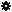﻿ Object Model: NurbsCurve.EvaluateNormalizedPosition

NurbsCurve.EvaluateNormalizedPosition

## NurbsCurve.EvaluateNormalizedPosition### Description

Returns a 3D vector containing the position, tangent vector in U direction, normal and bi-normal at a given normalized U value.

### C# Syntax

 ```Object NurbsCurve.EvaluateNormalizedPosition( Double in_dUValueNormalized ); ```

### Scripting Syntax

 ```oArray = NurbsCurve.EvaluateNormalizedPosition( UValueNormalized ); ```

### Return Value

A 1-dimensional Array containing the position, tangent vector, normal and bi-normal.

### Parameters

Parameter Type Description
UValueNormalized Double The normalized UValue(0.0 to 1.0) at which we want to evaluate the curve.

### Examples

#### VBScript Example

 ```set oRoot = Application.ActiveProject.ActiveScene.Root set oArc = oRoot.AddGeometry( "Arc", "NurbsCurve" ) aValues = oArc.ActivePrimitive.Geometry.Curves(0).EvaluateNormalizedPosition( 0.5 ) set oPosition = aValues(0) logmessage "The position at 0.5 is x :" & oPosition.x & " y: " & oPosition.y & " z: " & oPosition.z set oUTangent = aValues(1) logmessage "The tangent in U at 0.5 is x :" & oUTangent.x & " y: " & oUTangent.y & " z: " & oUTangent.z set oNormal = aValues(2) logmessage "The normal at 0.5 is x :" & oNormal.x & " y: " & oNormal.y & " z: " & oNormal.z set oBiNormal = aValues(3) logmessage "The bi-normal at 0.5 is x :" & oBiNormal.x & " y: " & oBiNormal.y & " z: " & oBiNormal.z ```